Journal homepage ISSN: 1303 - 2968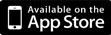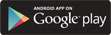©Journal of Sports Science and Medicine (2006) 05, 567 - 574 Research article Reducing the Likelihood of Long Tennis Matches Tristan Barnett1,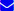, Brown Alan1, Graham Pollard2 Author Information 1 Swinburne University, Melbourne, VIC, Australia 2 University of Canberra, Canberra, ACT, Australia Tristan Barnett✉ Swinburne University, Melbourne, VIC, Australia.Email: strategicgames@hotmail.com Publish Date Received: -- Accepted: -- Published (online): 15-12-2006 × Tristan Barnett, Brown Alan, Graham Pollard. (2006) Reducing the Likelihood of Long Tennis Matches. Journal of Sports Science and Medicine (05), 567 - 574.  Email link to this article × Tristan Barnett, Brown Alan, Graham Pollard. (2006) Reducing the Likelihood of Long Tennis Matches. Journal of Sports Science and Medicine (05), 567 - 574. Your name: Your E-mail: Recipient’s E-mail:
 Abstract Introduction Methods Results Discussion Author biography References

ABSTRACT

Long matches can cause problems for tournaments. For example, the starting times of subsequent matches can be substantially delayed causing inconvenience to players, spectators, officials and television scheduling. They can even be seen as unfair in the tournament setting when the winner of a very long match, who may have negative aftereffects from such a match, plays the winner of an average or shorter length match in the next round. Long matches can also lead to injuries to the participating players. One factor that can lead to long matches is the use of the advantage set as the fifth set, as in the Australian Open, the French Open and Wimbledon. Another factor is long rallies and a greater than average number of points per game. This tends to occur more frequently on the slower surfaces such as at the French Open. The mathematical method of generating functions is used to show that the likelihood of long matches can be substantially reduced by using the tiebreak game in the fifth set, or more effectively by using a new type of game, the 50-40 game, throughout the match.

Key words: Tennis, scoring systems, sport, generating functions, long tennis matches

Key Points
• The cumulant generating function has nice properties for calculating the parameters of distributions in a tennis match
• A final tiebreaker set reduces the length of matches as currently being used in the US Open
• A new 50-40 game reduces the length of matches whilst maintaining comparable probabilities for the better player to win the match.

 INTRODUCTION

In recent years there have been a number of grand slam matches decided in long fifth sets. In the third round of the 2000 Wimbledon mens singles, Philippoussis defeated Schalken 20-18 in the fifth set. Ivanisevic defeated Krajicek 15-13 in the semi-finals of Wimbledon in 1998. In the quarter-finals of the 2003 Australian Open mens singles, Andy Roddick defeated Younes El Aynaoui 21-19 in the fifth set, a match taking 83 games to complete and lasting a total duration of 5 hours. The night session containing this long match required the following match to start at 1 am. Long matches require rescheduling of following matches, and also create scheduling problems for media broadcasters. They arise because of the advantage set, which gives more chance of winning to the better player (Pollard and Noble, 2002), but has no upper bound on the number of games played. It may be in the interests of broadcasters and tournament organizers to decrease the likelihood of long tennis matches occurring.

Pollard, 1983 calculated the mean and variance of the duration of a best-of-three sets match of classical and tiebreaker tennis by using the probability generating function. It is well established that the mean and standard deviation completely describe the normal distribution. When a distribution is not symmetrical about the mean, the coefficients of skewness and kurtosis, as defined in Stuart and Ord, 1987, are important to graphically interpret the shape of the distribution. This commonly has been done by using the probability or moment generating function. The cumulant generating function (taking the natural logarithm of the moment generating function), can also be used to calculate the parameters of the distribution in a tennis match. The cumulant generating function is particularly useful for calculating the parameters of distributions for the number of points in a tiebreaker match, since the critical property of cumulant generating functions is that they are additive for linear combinations of independent random variables. The layout of this paper is as follows. For convenience of the less mathematically inclined we defer the presentation of the mathematics of generating functions applied to tennis till Section 3. Instead we will begin in Section 2 with a discussion on several aspects of long matches, relying on graphical results to advance our arguments as to how they might be curtailed. We aim to show that the likelihood of long matches can be substantially reduced by using the tiebreak game in the fifth set, or more effectively by the use of a new type of game, the 50-40 game (Pollard and Noble, 2004), throughout the match. In Section 4 we make some concluding remarks.

Discussion of the Problem (Using Graphical Results)

Up until 1970 (approx), all tennis sets were played as advantage sets, where to win a set a player must reach at least 6 games and be ahead by at least 2 games. The tiebreaker game was introduced to shorten the length of matches. A tiebreaker game is played when the set score reaches 6-games all. However in three of the four grand slams (Australian Open, French Open and Wimbledon), an advantage set is still played in the deciding fifth set. Figure 1 represents a comparison of a match with 5 advantage sets (5adv), 5 tiebreaker sets (5tie) and 4 tiebreaker sets with a deciding advantage set (4tie1adv). The probability of each player winning a point on serve is given as 0.6 to represent averages in men’s tennis. The long tail given by the match with 5adv gives an indication as to why the tiebreaker game was introduced to the tennis scoring system. It is well known that the dominance of serve in men’s tennis has increased since the introduction of the tiebreaker game. This creates a problem when two big servers meet in a grand slam event where the deciding fifth set is played as an advantage set.

Figure 2 represents a match with 4tie1adv for different values of players winning points on serve. It shows that for two strong servers winning 0.7 of points on serve, there is a long tail in the number of points played. In comparison with Figure 3, which represents a match with 5tie, the tail is substantially reduced for two players winning 0.7 of points on serve.

Figure 4 represents a match with 5 tiebreaker sets, where a standard ‘deuce’ game is replaced by a 50-40 game. It shows an even greater improvement to reducing the number of points played in a match compared to Figure 3. In the 50-40 game the server has to win the standard 4 points, while the receiver only has to win 3 points. Such a game requires at most 6 points.

The Mathematics of Generating FunctionsModelling a Tennis Match
Forward Recursion

The state of a tennis match between two players is represented by a scoreboard. The scoreboard shows the points, games and sets won by each player, and is updated after each point has been played. It is assumed that the conditional probability of the server winning the point depends only on the data shown on the scoreboard. This enables the progress of the match to be modelled using forward recursion. An additional assumption is that the probabilities of each player winning a point on his own service remain constant throughout the match.

Development of Generating Functions of Distributions

The forward recursion enables the probabilities of various possible scoreboards to be calculated. These probabilities can be collected in the form of probability generating functions, or moment generating functions (using the transformation v = eu).

Lemma: If X and Y are independent random variables and Z = X + Y then: mZ(t) = mX(t) * mY(t).

It becomes convenient at times to take logarithms, and work in terms of cumulant generating functions, since KZ(t) = KX(t) + KY(t).

The higher order cumulants depend on powers of the scale for the random variable, and for the purposes of communication it is useful to transform them into non-dimensional statistics (i.e. numbers) such as the coefficients of variation, skewness and kurtosis.

The Inversion of the Cumulants Using Normal Power Approximation

This gives a continuous approximation to a discrete distribution (Pesonen, 1975). The formula is asymptotic and works reasonably well for unimodal distributions with the coefficient of skewness less than 2 and the coefficient of kurtosis less than 6. i.e. tails die off at least as fast as the exponential distribution.

The Number of Points in a Game

Let X be a random variable of the number of points played in a game. Let fpgA(x) represent the distribution of the number of points played in a game for player A serving, where fpgA (x) = P(X = x). This gives the following:

Croucher, 1986 gives algebraic expressions for calculating NpgA(a,b).

Let m(t) denote the moment generating function X. Generating functions can be used to describe a distribution, such as f pgA(x) for all x. It is well established (Stuart and Ord,1987) that the mean, variance, coefficient of skewness and coefficient of kurtosis of X can be obtained from generating functions.

The moment generating function for the number of points in a game for player A serving, mpgA(t), becomes:

The mean number of points in a game MpgA, with the associated variance VpgA are calculated from the moment generating function using Mathematica and given as:

Similar expressions can be obtained for the coefficient of skewness SpgA, and the coefficient of kurtosis KpgA.

Let UpgA represent the standard deviation of the number of points in a game for player A serving. Let CpgA represent the coefficient of variation of the number of points in a game for player A serving. It follows that UpgA = √V pgA and CpgA = UpgA / MpgA.

Table 1 represents MpgA, UpgA, CpgA, SpgA and KpgA for different values of pA. Notice that the mean and standard deviation are greatest when pA = 0.50, but the coefficients of skewness and kurtosis are greatest when pA approaches 1 or 0. The generating functions to follow are for player A serving first in the tiebreaker game or set.

The moment generating function for the number of points in a tiebreaker game, mpgTA(t) becomes:

The moment generating functions for the number of games in a tiebreaker set, mgsTA(t) and advantage set, mgsA(t) become:

The Number of Points in a Set
The Parameters of Distributions of the Number of Points in a Set

Let mpgA+(t) and mpgA-(t) be the moment generating functions of the number of points in a game when player A wins and loses a game on serve respectively. Let mpgB+(t) and mpgB-(t) be the moment generating functions of the number of points in a game when player B wins and loses a game on serve respectively. Let s(c,d) be the moment generating function of the number of points in a set conditioned on reaching game score (c,d). It can be shown that

Similar conditional moment generating functions can be obtained for reaching all score lines (c,d) in a set. The moment generating function for the number of points in a tiebreaker set becomes:

A similar moment generating function can be obtained for the number of points in an advantage set.

Let MpsA, UpsA, CpsA, SpsA and KpsA represent the mean, standard deviation, and coefficients of variation, skewness and kurtosis for the number of points in an advantage set. Let MpsTA , UpsTA, CpsTA, SpsTA and KpsTA represent the mean, standard deviation, and coefficients of variation, skewness and kurtosis for the number of points in a tiebreaker set. Table 2 represents MpsA, UpsA, CpsA, SpsA, KpsA, MpsTA, UpsTA, CpsTA, SpsTA and KpsTA for different values of pA and pB. The table covers values in the interval 0.50 ≤ pA ≤ pB ≤ 0.75 as this is the main area of interest for men’s tennis. It can be observed that: MpsA > MpsTA, UpsA > UpsTA, CpsA > CpsTA, SpsA > SpsTA and KpsA > KpsTA .

The mean number of points in a set is affected by the mean number of points in a game and the mean number of games in a set. The mean number of points in a game is greatest when pA or pB = 0.50. For a tiebreaker set, when pA = pB = 0.50, MpgA = MpgB = 6.75, MgsTA =9.66 and MpsTA = 65.83. When pA = pB = 0.70, MpgA = MpgB = 5.83, MgsTA = 10.94 and MpsTA = 66.22. For this latter case, even though the mean length of games is shorter, the mean number of points in a tiebreaker set overall is greater since more games are expected to be played. Both players have a 0.90 probability of holding serve, which means that very few breaks of serve will occur and there is a 0.38 probability of reaching a tiebreaker. This is further exemplified in an advantage set, where for pA = pB = 0.70, MpsA = 86.43. This is also highlighted by the coefficients of variation, skewness and kurtosis being much greater for an advantage set, compared to a tiebreaker set, when pA and pB are both “large”.

Approximating the Parameters of Distributions of the Number of Points in a Set

The moment generating function for the number of points in an advantage set mpsA(t), when pA = 1 - pB, becomes:

Taking the natural logarithm of the moment generating function gives an alternative generating function known as the cumulant generating function. Let κpgA(t)=ln[mpgA(t)] represent the cumulant generating function for the number of points in a game. This relationship can be inverted to give mpgA (t) = exp(κpgA(t)).

The moment generating function, mpsA (t), can be written as:

This can be expressed as:

Similarly, the following result is established for mpsTA(t), when pA = 1 - pB:

Notice the last term does not vanish due to the difference in the scoring system for a tiebreaker game compared with a regular game. Equations (1) and (2) can be used to obtain approximate results for the parameters of distributions for the number of points in a set, when pA is not equal to 1 - pB.

The Number of Points in a Match

From this point an advantage match is considered as a match where the first four sets played are tiebreaker sets and the fifth set is an advantage set.

The moment generating functions for the number of points in an advantage and tiebreaker match, mpm(t) and mpmT(t), when pA = 1 - pB become:

The following approximation results can be established for the number of points in a match, similar to the approximation results established for the number of points in a set:

Approximation results for distributions of points in a match, could also be established for tennis doubles by using the above results established for singles. The probability of a team winning a point on serve is estimated by the averages of the two players in the team.

When pA = 1-pB, the distribution of number of points played each set if player A serves first in the set, is equal to the number of points played each set if player B serves first in the set. This leads to the following result:

The number of points played each set in a match are independent, if pA = 1 - pB.

Suppose Z=X+Y, where X and Y are independent, then it is well known that mZ(t) = E[eZt]=E[eXt]E[eYt]=mX(t)mY(t). By taking logarithms it follows that κZ(t) = κX(t) + κY (t).

An extension of this property of cumulants is given by the following theory (Brown, 1977) and can be applied to points in a tiebreaker match when the number of points played each set in a match are independent. When the independence assumption fails to hold the theory remains approximately correct according to the approximation result established for points in a tiebreaker match.

Theorem

If Z = X1 + X2 +……… + XN where Xi are i.i.d. then κZ(t) = κN(κX(t)). Taking the derivatives of the result and setting t = 0 gives the following useful results in terms of cumulants:

For example the mean number of points in a tiebreaker match, MpmT, with the associated variance, VpmT, can be calculated from the cumulant generating function as:

Let Mpm, Upm, Cpm, Spm and Kpm represent the mean, standard deviation, and coefficients of variation, skewness and kurtosis for the number of points in an advantage match. Let MpmT, UpmT, CpmT, SpmT and KpmT represent the mean, standard deviation, and coefficients of variation, skewness and kurtosis for the number of points in a tiebreaker match. Tables 3 and 4 represent the exact parameters of the distributions for an advantage and tiebreaker match for different values of pA and pB. The results agree with Pollard (1983) for a best-of-three sets tiebreaker match. It shows that the mean, standard deviation, coefficients of variation, skewness and kurtosis of the number of points played are greater for an advantage match, compared to a tiebreaker match. Also included in the tables are the probabilities of the match lasting for at least n points, represented by P(n) for an advantage match and Q(n) for a tiebreaker match. These probabilities were calculated using the NP-expansion technique (Pesonen, 1975). Notice that when pA and pB become “large”, the probability of playing at least 400 points in an advantage match is considerably greater than for a tiebreaker match. This is some justification as to why an advantage match can seemingly never end with two strong servers.

Table 5 represents the exact parameters of distributions for a tiebreaker and an advantage match using 50-40 games, along with the probability of a match going beyond 300 points. For an extreme case, when pA = pB = 0.75, the probability of an advantage match going beyond 300 points is 0.06. In comparison to Tables 3 and 4">4, the probability of an advantage or tiebreaker match going beyond 300 points is 0.38. This shows that replacing standard ‘deuce’ games with 50-40 games, substantially decreases the likelihood of long matches occurring.

It is often the case that by shortening the length of matches, decreases the probability of winning for the better player. However this is not necessarily the case as shown by replacing standard ‘deuce’ games with 50-40 games. Table 6 represents the probabilities of winning under four different scoring systems, for different values of pA and pB. Notice when pA=0.75 and pB = 0.70, the probability of player A (the stronger player) winning using 50-40 games is greater than using standard ‘deuce’ games.

Conclusions

The mathematical methods of generating functions have been used to calculate the parameters of distributions of the number of points in a tennis match. The results show that the likelihood of long matches can be substantially reduced by using the tiebreak game in the fifth set, or more effectively by using the 50-40 game throughout the match.

We used the number of points played in a match as a measure of its length. This measure is related to the time duration of the match and avoids the complications of delays between points, at change of serve, at change of end, injury time and weather delays. Further work could involve calculating the time duration of a match from the results presented in this paper. This could then be used to calculate the probabilities of the match going beyond a given amount of time. This would provide commentators and tournament officials with very useful information on when the match is going to finish.

AUTHOR BIOGRAPHYTristan Barnett Employment: Gaming Mathematician. Degree: PhD from Swinburne University Research interests: sports modelling, mathematics of gambling games. E-mail: strategicgames@hotmail.comBrown Alan Employment: Retired Actuary. Degree: M Sc Research interests: operational research, health insurance. E-mail: abrown@labyrinth.net.auGraham Pollard Employment: Emeritus Professor, University of Canberra. Degree: PhD in Statistics from the Australian National University Research interests: Probability applications in sports scoring systems and in assessment, optimal learning. E-mail: graham@foulsham.com.au

REFERENCES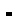Barnett T., Clarke S.R. (2005) Combining player statistics to predict outcomes of tennis matches. IMA Journal of Management Mathematics 16, 113-120.Brown A. (1977) Cumulants of convolution-mixed distributions. Astin Bulletin 9, 59-63.Croucher J.S. (1986) The conditional probability of winning games of tennis. Research Quarterly for Exercise and Sport 57, 23-26.Pesonen E. (1975) NP-technique as a tool in decision making. Astin Bulletin 8, 359-363.Pollard G.H. (1983) An analysis of classical and tie-breaker tennis. Australian Journal of Statistics 25, 496-505.Pollard G.H., Noble K., Cohen G., Langtry T. (2002) Proceedings of the 6th Australasian Conference on Mathematics and Computers in Sport. The characteristics of some new scoring systems in tennis. Queensland, Australia. Bond University.Pollard G.H., Noble K., Morton H., Ganesalingam S. (2004) Proceedings of 7th Australasian Conference on Mathematics and Computers in Sport. The benefits of a new game scoring system in tennis the 50-40 game. Palmerston Norton, New Zealand. Massey University.Stuart A., Ord J.K. (1987) Kendall’s advanced theory of statistics. London. Charles Griffin & Company Limited.

 Home Issues About Authors Contact Current Editorial board Authors instructions Email alerts In Press Mission For Reviewers Archive Scope Supplements Statistics Most Read Articles Most Cited Articles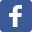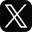This work is licensed under a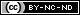Creative Commons Attribution-NonCommercial-NoDerivatives 4.0 International License.